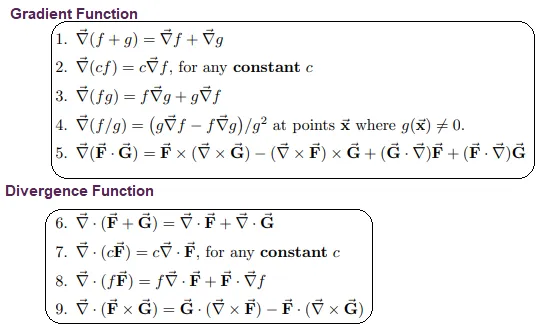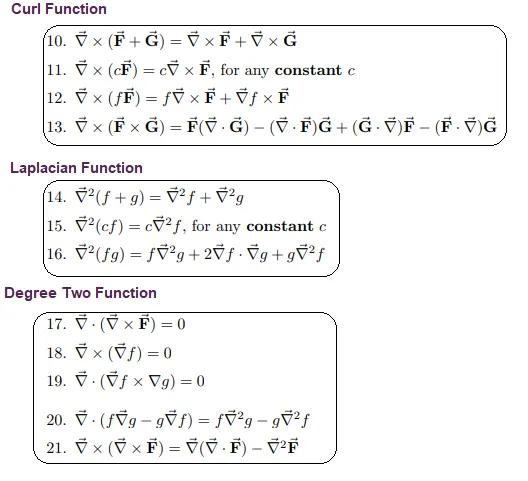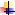# Vector calculus

In Mathematics, Calculus is a branch that deals with the study of the rate of change of a function. Calculus plays an integral role in many fields such as Science, Engineering, Navigation, and so on. Generally, calculus is used to develop a Mathematical model to get an optimal solution. We know that calculus can be classified into two different types, such as differential calculus and integral calculus. But we might not be aware of vector calculus. In this article, we are going to discuss the definition of vector calculus, formulas, applications, line integrals, the surface integrals, in detail.

Vector Calculus Definition

Vector Calculus, also known as vector analysis, deals with the differentiation and integration of vector field, especially in the three-dimensional Euclidean space. Vector fields represent the distribution of a vector to each point in the subset of space. In Euclidean Space, the vector field on a domain is represented as a vector-valued function that compares the n-tuple of real numbers to each point on the domain. Vector analysis is an analysis which deals with the quantities that have both magnitude and direction. Vector calculus deals with two integrals such as line integrals and surface integrals.

Line Integral

In Vector Calculus, a line integral of a vector field is defined as an integral of some function along a curve. In simple words, a line integral is an integral in which the function to be integrated is calculated along with a curve. We can integrate some specific type of vector-valued functions along with a curve. For example, we can also integrate a scalar-valued function along a curve. Sometimes, a line integral is also known as a path integral, or curve integral or curvilinear integrals.

Surface Integral

In calculus, a surface integral is defined as a generalisation of several integrals to the integrations over surfaces. It means that we can think about the double integral related to the line integral. For any given surface, we can integrate a scalar field over a surface or a vector field over a surface.

Vector Calculus Formulas

Some of the important vector calculus formulas are given below:

From fundamental theorems, we take,

F(x, y, z) = P(x, y, z)i + Q(x, y, z)j + R(x, y, z)k

Fundamental Theorem of Line Integral

If F = ∇f and curve C has endpoints A and B, then

∫c F. dr= f(B) − f(A).

Circulation-Curl Form

Green’s Theorem

∫∫D (∂Q /∂x) – (∂P/ ∂y)dA = ∮C F· dr

Stokes’ Theorem

∫∫D ∇ × F · n dσ = ∮C F· dr, where C is the edge curve of S

Flux – Divergence Form

Green’s Theorem

∫∫D ∇· F dA = ∮C F · n ds

Divergence Theorem

∫∫∫D ∇· F dV = ∯S F · n dσ

Vector Calculus Identities

The list of Vector Calculus identities are given below for different functions such as Gradient function, Divergence function, Curl function, Laplacian function, and degree two functions.

Vector Calculus Applications

Vector Calculus plays an important role in the different fields such as;Used in heat transferNavigationSportsThree-dimensional geometryPartial differential equation